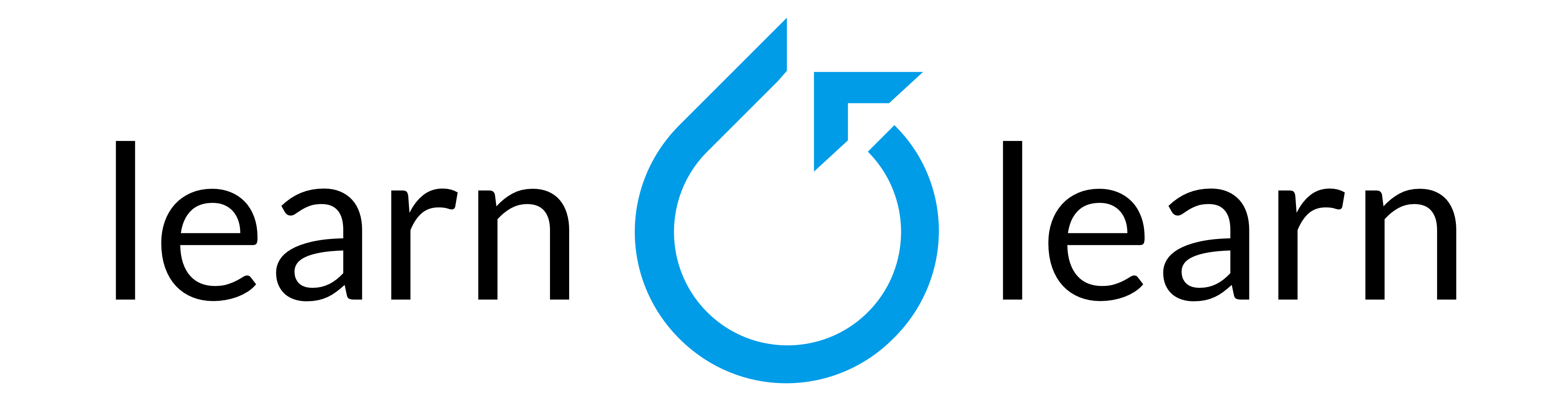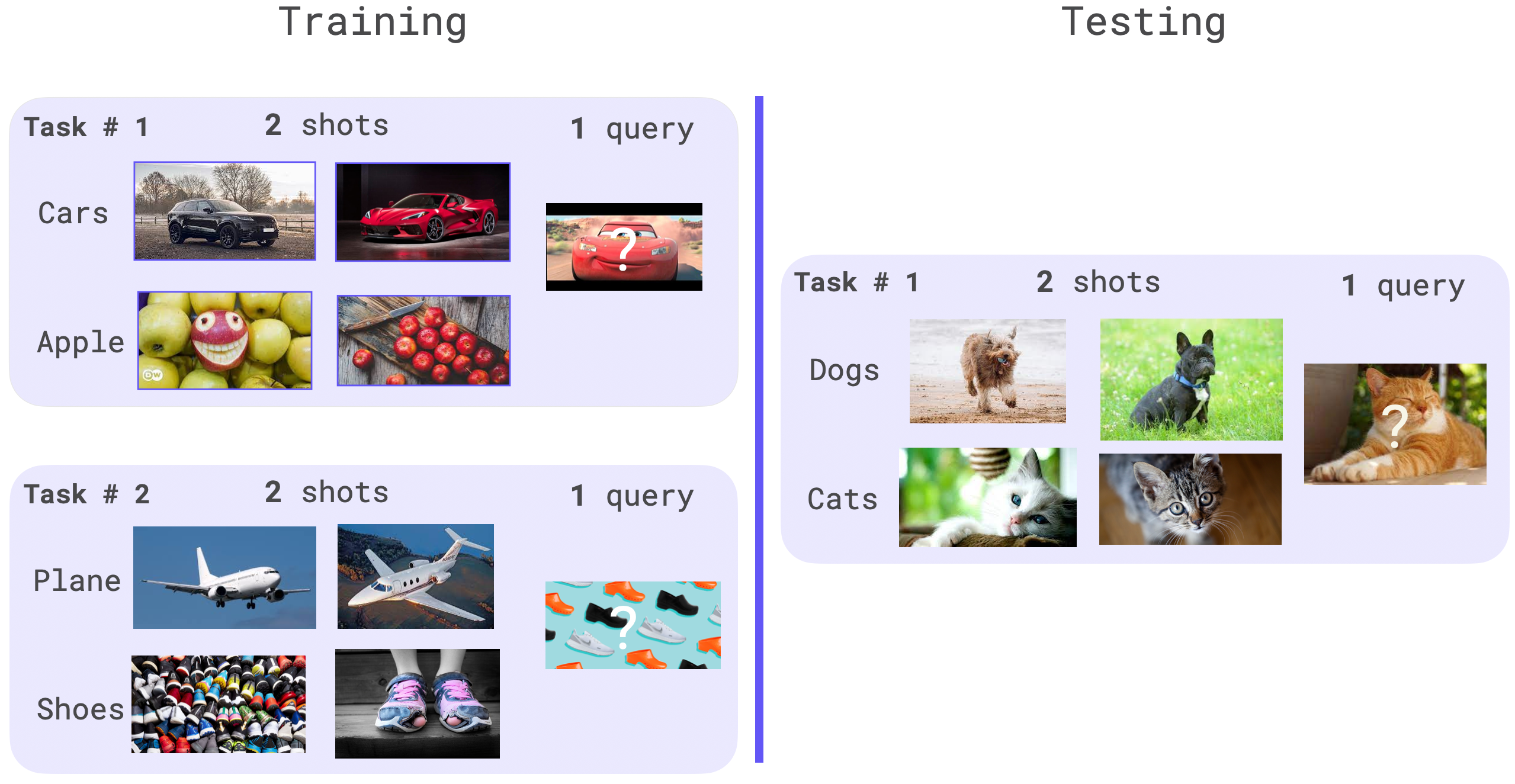Shortcuts

Learn2Learn¶

Learn2Learn is a software library for meta-learning research by Sébastien M. R. Arnold and al. (Aug 2020)What is Meta-Learning and why you should care?¶

Humans can distinguish between new objects with little or no training data, However, machine learning models often require thousands, millions, billions of annotated data samples to achieve good performance while extrapolating their learned knowledge on unseen objects.

A machine learning model which could learn or learn to learn from only few new samples (K-shot learning) would have tremendous applications once deployed in production. In an extreme case, a model performing 1-shot or 0-shot learning could be the source of new kind of AI applications.

Meta-Learning is a sub-field of AI dedicated to the study of few-shot learning algorithms. This is often characterized as teaching deep learning models to learn with only a few labeled data. The goal is to repeatedly learn from K-shot examples during training that match the structure of the final K-shot used in production. It is important to note that the K-shot example seen in production are very likely to be completely out-of-distribution with new objects.

How does Meta-Learning work?¶

In meta-learning, the model is trained over multiple meta tasks. A meta task is the smallest unit of data and it represents the data available to the model once in its deployment environment. By doing so, we can optimise the model and get higher results.For image classification, a meta task is comprised of shot + query elements for each class. The shots samples are used to adapt the parameters and the queries ones to update the original model weights. The classes used in the validation and testing shouldn’t be present within the training dataset, as the goal is to optimise the model performance on out-of-distribution (OOD) data with little label data.

When training the model with the meta-learning algorithm, the model will average its gradients over meta_batch_size meta tasks before performing an optimizer step. Traditionally, an meta epoch is composed of multiple meta batch.

Use Meta-Learning with Flash¶

With its integration within Flash, Meta Learning has never been simpler. Flash takes care of all the hard work: the tasks sampling, meta optimizer update, distributed training, etc…

Note

The users requires to provide a training dataset and testing dataset with no overlapping classes. Flash doesn’t support this feature out-of-the box.

Once done, the users are left to play the hyper-parameters associated with the meta-learning algorithm.

Here is an example using miniImageNet dataset containing 100 classes divided into 64 training, 16 validation, and 20 test classes.

import warnings

import kornia.augmentation as Ka
import kornia.geometry as Kg
import learn2learn as l2l
import torch
import torchvision
from torch import nn

import flash
from flash.core.data.data_source import DefaultDataKeys
from flash.core.data.transforms import ApplyToKeys, kornia_collate
from flash.image import ImageClassificationData, ImageClassifier

warnings.simplefilter("ignore")

train_transform = {
"to_tensor_transform": nn.Sequential(
ApplyToKeys(DefaultDataKeys.INPUT, torchvision.transforms.ToTensor()),
ApplyToKeys(DefaultDataKeys.TARGET, torch.as_tensor),
),
"post_tensor_transform": ApplyToKeys(
DefaultDataKeys.INPUT,
Kg.Resize((196, 196)),
# SPATIAL
Ka.RandomHorizontalFlip(p=0.25),
Ka.RandomRotation(degrees=90.0, p=0.25),
Ka.RandomAffine(degrees=1 * 5.0, shear=1 / 5, translate=1 / 20, p=0.25),
Ka.RandomPerspective(distortion_scale=1 / 25, p=0.25),
# PIXEL-LEVEL
Ka.ColorJitter(brightness=1 / 30, p=0.25),  # brightness
Ka.ColorJitter(saturation=1 / 30, p=0.25),  # saturation
Ka.ColorJitter(contrast=1 / 30, p=0.25),  # contrast
Ka.ColorJitter(hue=1 / 30, p=0.25),  # hue
Ka.RandomMotionBlur(kernel_size=2 * (4 // 3) + 1, angle=1, direction=1.0, p=0.25),
Ka.RandomErasing(scale=(1 / 100, 1 / 50), ratio=(1 / 20, 1), p=0.25),
),
"collate": kornia_collate,
"per_batch_transform_on_device": ApplyToKeys(
DefaultDataKeys.INPUT,
Ka.RandomHorizontalFlip(p=0.25),
),
}

# construct datamodule
datamodule = ImageClassificationData.from_tensors(
train_data=train_dataset.x,
train_targets=torch.from_numpy(train_dataset.y.astype(int)),
val_data=val_dataset.x,
val_targets=torch.from_numpy(val_dataset.y.astype(int)),
test_data=test_dataset.x,
test_targets=torch.from_numpy(test_dataset.y.astype(int)),
num_workers=4,
train_transform=train_transform,
)

model = ImageClassifier(
backbone="resnet18",
training_strategy="prototypicalnetworks",
training_strategy_kwargs={
"epoch_length": 10 * 16,
"meta_batch_size": 4,
"ways": datamodule.num_classes,
"shots": 1,
"test_ways": 5,
"test_shots": 1,
"test_queries": 15,
},
optimizer_kwargs={"lr": 0.001},
)

trainer = flash.Trainer(
max_epochs=200,
gpus=2,
accelerator="ddp_shared",
precision=16,
)
trainer.finetune(model, datamodule=datamodule, strategy="no_freeze")

You can read their paper Learn2Learn: A Library for Meta-Learning Research.

And don’t forget to cite Learn2Learn repository in your academic publications. Find their Biblex on their repository.

Built with Sphinx using a theme provided by Read the Docs.
Versions
latest
stable
0.5.1
0.5.0
0.4.0
0.3.2
0.3.1
0.3.0
0.2.3
0.2.2
0.2.1
0.2.0
0.1.0post1
docs-fix_build